In this article, we will share MP Board Class 10th Maths Book Solutions Chapter 10 Circles Ex 10.2 Pdf, These solutions are solved subject experts from the latest edition books.

## MP Board Class 10th Maths Solutions Chapter 10 Circles Ex 10.2

In questions 1 to 3, choose the correct option and give justification.

Question 1.
From a point Q, the length of the tangent to a circle is 24 cm and the distance of Q from the centre is 25 cm. The radius of the circle is
(A) 7 cm
(B) 12 cm
(C) 15 cm
(D) 24.5 cm
Solution:
(A): ∵ QT is a tangent to the circle at T and OT is radius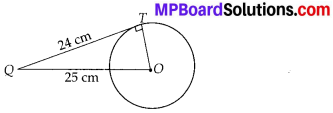∴ OT⊥QT
Also, OQ = 25 cm and QT = 24 cm
∴ Using Pythagoras theorem, we get
OQ2 = QT2 + OT2
⇒ OT2 = OQ2 – QT2 = 252 – 242 = 49
⇒ OT = 7
Thus, the required radius is 7 cm.

Question 2.
In figure, if TP and TQ are the two tangents to a circle with centre 0 so that ∠POQ =110°, then ∠PTQ is equal to
(A) 60°
(B) 70°
(C) 80°
(D) 90°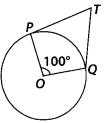Solution:
(B): TQ and TP are tangents to a circle with centre O and ∠POQ = 110°
∴ OP⊥PT and OQ⊥QT
⇒ ∠OPT = 90° and ∠OQT = 90°
Now, in the quadrilateral TPOQ, we get
∠PTQ + 90° + 110° + 90° = 360° [Angle sum property of a quadrilateral]
⇒ ∠PTQ + 290° = 360°
⇒ ∠PTQ = 360° – 290° = 70°Question 3.
If tangents PA and PB from a point P to a circle with centre O are inclined to each other at angle of 80°, then ∠POA is equal to
(A) 50°
(B) 60°
(C) 70°
(D) 80°
Solution:
(A) : Since, O is the centre of the circle and two tangents from P to the circle are PA and PB.
∴ OA⊥AP and OB⊥BP
⇒ ∠OAP = ∠OBP = 90°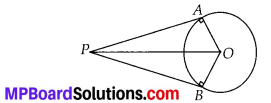Now, in quadrilateral PAOB, we have
∠BPA + ∠PAO + ∠AOB + ∠OBP = 360°
⇒ 80° + 90° + ∠AOB + 90° = 360°
⇒ 260° + ∠AOB = 360°
⇒ ∠AOB = 360° – 260° ⇒ ∠AOB = 100°
In right ∆OAP and right ∆OBP, we have
OP = OP [Common]
∠OAP = ∠OBP [Each 90°]
OA = OB [Radii of the same circle]
∴ ∆OAP ≅ ∆OBP [By RHS congruency]
⇒ ∠POA = ∠POB [By CPCT]
∴ ∠POA = $$\frac{1}{2}$$ ∠AOB = $$\frac{1}{2}$$ × 100° = 50°

Question 4.
Prove that the tangents drawn at the ends of a diameter of a circle are parallel.
Solution:
In the figure, PQ is diameter of the given circle and O is its centre.
Let tangents AB and CD be drawn at the end points of the diameter PQ.
Since, the tangents at a point to a circle is perpendicular to the radius through the point.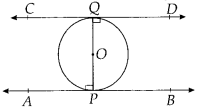∴ PQ⊥AB
⇒ ∠APQ = 90°
And PQ⊥CD
⇒ ∠PQD = 90° ⇒ ∠APQ = ∠PQD
But they form a pair of alternate angles.
∴ AB || CD
Hence, the two tangents are parallel.

Question 5.
Prove that the perpendicular at the point of contact to the tangent to a circle passes through the centre.
Solution:
In the figure, the centre of the circle is O and tangent AB touches the circle at P. If possible, let PQ be perpendicular to AB such that it is not passing through O.
Join OP.
Since, tangent at a point to a circle is perpendicular to the radius through that point.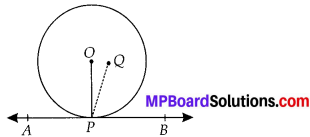∴ OP⊥AB
⇒ ∠OPB = 90° ……….. (1)
But by construction, PQ⊥AB
⇒ ∠QPB = 90° ………….. (2)
From (1) and (2),
∠QPB = ∠OPB
which is possible only when O and Q coincide. Thus, the perpendicular at the point of contact to the tangent to a circle passes through the centre.

Question 6.
The length of a tangent from a point A at distance 5 cm from the centre of the circle is 4 cm. Find the radius of the circle.
Solution:
∵ The tangent to a circle is perpendicular to the radius through the point of contact.
∴ ∠OTA = 90°
Now, in the right ∆OTA, we have
OA2 = OT2 + AT2 [Pythagoras theorem]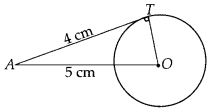⇒ OT2 = 52 – 42
⇒ OT2 = (5 – 4)(5 + 4)
⇒ OT2 = 1 × 9 = 9 = 32
⇒ OT = 3
Thus, the radius of the circle is 3 cm.Question 7.
Two concentric circles are of radii 5 cm and 3 cm. Find the length of the chord of the larger circle which touches the smaller circle.
Solution:
In the figure, O is the common centre, of the given concentric circles.
AB is a chord of the bigger circle such that it is a tangent to the smaller circle at P.
Since, OP is the radius of the smaller circle.
∴ OP⊥AB ⇒ ∠APO = 90°
Also, radius perpendicular to a chord bisects the chord.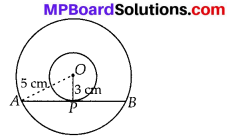∴ OP bisects AB
⇒ AP = $$\frac{1}{2}$$ AB
Now, in right ∆APO,
OA2 = AP2 + OP2
⇒ 52 = AP2 + 32 ⇒ AP2 = 52 – 32
⇒ AP2 = 42 ⇒ AP = 4 cm
⇒ $$\frac{1}{2}$$ AB = 4 ⇒ AB = 2 × 4 = 8 cm
Hence, the required length of the chord AB is 8 cm.

Question 8.
A quadrilateral ABCD is drawn to circumscribe a circle (see figure).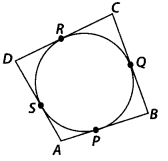Prove that AB + CD = AD + BC
Solution:
Since, the sides of quadrilateral ABCD, i.e., AB, BC, CD and DA touches the circle at P, Q, R and S respectively, and the lengths of two tangents to a circle from an external point are equal.
∴ AP = AS, BP = BQ,
DR = DS and CR = CQ
(AP + BP) + (CR + RD) = (BQ + QQ) + (DS + SA)
⇒ AB + CD = BC + DA

Question 9.
In figure, XY and X’Y’ are two parallel tangents to a circle with centre O and another tangent AB with point of contact C intersecting XY at A and X’Y’ at B.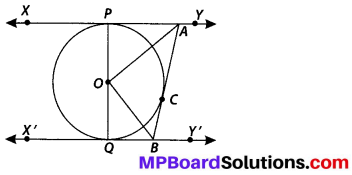Prove that ∠AOB = 90°.
Solution:
∵ The tangents drawn to a circle from an external point are equal.
∴ AP = AC ……… (1)
Join OC.
In ∆PAO and ∆CAO, we have
AO = AO [Common]
OP = OC [Radii of the same circle]
AP = AC [From (1)]
⇒ ∆PAO ≅ ∆CAO [SSS congruency]
∴ ∠PAO = ∠CAO
⇒ ∠PAC = 2∠CAO …………. (2)
Similarly, ∠CBQ = 2∠CBO ……………… (3)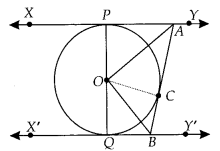Again, we know that sum of internal angles on the same side of a transversal is 180°.
∴ ∠PAC + ∠CBQ = 180°
2∠CAO + 2∠CBO = 180° [From (2) and (3)]
⇒ ∠CAO + ∠CBO = $$\frac{180^{\circ}}{2}$$ = 90° …………… (4)
Also, in ∆AOB,
∠BAO + ∠OBA + ∠AOB = 180° [Sum of angles of a triangle]
⇒ ∠CAO + ∠CBO + ∠AOB = 180°
⇒ 90° + ∠AOB = 180° [From (4)]
⇒ ∠AOB = 180° – 90°
⇒ ∠AOB = 90°

Question 10.
Prove that the angle between the two tangents drawn from an external point to a circle is supplementary to the angle subtended by the line-segment joining the points of contact at the centre.
Solution:
Let PA and PB be two tangents drawn from an external point P to a circle with centre O.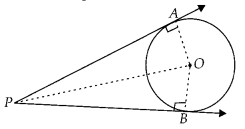Now, in right ∆OAP and right ∆OBP, we have
PA = PB [Tangents to circle from an external point]
OA = OB [Radii of the same circle]
OP = OP [Common]
⇒ ∆OAP ≅ ∆OBP [By SSS congruency]
∴ ∠OPA = ∠OPB [By CPCT]
and ∠AOP = ∠BOP
⇒ ∠APB = 2∠OPA and ∠AOB = 2∠AOP
In right ∆OAP,
∠AOP + ∠OPA + ∠PAO = 180°
⇒ ∠AOP = 180° – 90° – ∠OPA
⇒ ∠AOP = 90° – ∠OPA
⇒ 2∠AOP = 180° – 2∠OPA
⇒ ∠AOB = 180° – ∠APB
⇒ ∠AOB + ∠APB = 180°Question 11.
Prove that the parallelogram circumscribing a circle is a rhombus.
Solution:
We have ABCD, a parallelogram which circumscribes a circle (i.e., its sides touch the circle) with centre O.
Since, tangents to a circle from an external point are equal in length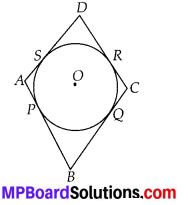∴ AP = AS
BP = BQ
CR = CQ
DR = DS
(AP + BP) + (CR + DR) = (AS + DS) + (BQ + CQ)
⇒ AB + CD = AD + BC
But AB = CD [Opposite sides of parallelogram]
∴ AB + CD = AD + BC ⇒ 2AB = 2BC
⇒ AB = BC
Similarly, AB = DA and DA = CD
Thus, AB = BC = CD = DA
Hence, ABCD is a rhombus.

Question 12.
A triangle ABC is drawn to circumscribe a circle of radius 4 cm such that the segments BD and DC into which BC is divided by the point of contact D are of lengths 8 cm and 6 cm respectively (see figure). Find the sides AB and AC.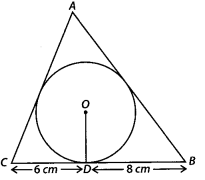Solution:
Here ∆ABC circumscribes the circle with centre O. Also, radius = 4 cm
Let AC and AB touches the circle at E and F, respectively and join OE and OF.
∵ The sides BC, CA and AB touches the circle at D, E and F respectively.
∴ BF = BD = 8 cm
[ ∵ Tangents to a circle from an external point are equal]
CD = CE = 6 cm
AF = AE = x cm (say)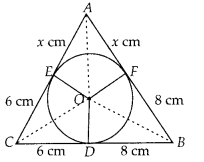∴ The sides of the ∆ABC are 14 cm, (x + 6) cm and (x + 8) cm
Perimeter of ∆ABC
= [14 + (x + 6) + (x + 8)] cm
= [14 + 6 + 8 + 2x] cm
= (28 + 2x) cm
⇒ Semi perimeter of ∆ABC,
s = $$\frac{1}{2}$$ [28 + 2x] cm = (14 + x) cm
∴ s – a = (14 + x) – (8 + x) = 6
s – b = (14 + x) – (14) = x
s – c = (14 + x) – (6 + x) = 8
where, a = AB, b = BC, c = AC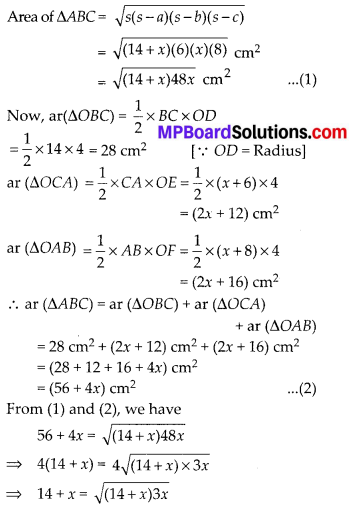Squaring both sides, we get
(14 + x)2 = (14 + x)3x
⇒ 196 + x2 + 28x = 42x + 3x2
⇒ 2x2 + 14x – 196 = 0
⇒ x2 + 7x – 98 = 0
⇒ (x – 7)(x + 14) = 0
⇒ x – 7 = 0 or x + 14 = 0
⇒ x = 7 or x = -14
But x = -14 is rejected.
∴ x = 7
Thus, AB = 8 + 7 = 15 cm, BC = 8 + 6 = 14 cm and CA = 6 + 7 = 13 cm

Question 13.
Prove that opposite sides of a quadrilateral circumscribing a circle subtend supplementary angles at the centre of the circle.
Solution:
We have a circle with centre O. A quadrilateral ABCD is such that the sides AB, BC, CD and DA touches the circle at P, Q, R and S respectively.
Join OP, OQ, OR and OS.
We know that two tangents drawn from an external point to a circle subtend equal angles at the centre.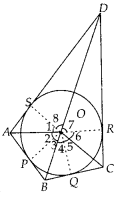∴ ∠1 = ∠2
∠3 = ∠4
∠5 = ∠6 and ∠7 = ∠8
Also, the sum of all the angles around a point is 360°.
∴ ∠1 + ∠2 + ∠3 + ∠4 + ∠5 + ∠6 + ∠7 + ∠8 = 360°
∴ 2(∠1 + ∠8 + ∠5 + ∠4) = 360°
⇒ (∠1 + ∠8 + ∠5 + ∠4) = 180° …………. (1)
and 2(∠2 + ∠3 + ∠6 + ∠7) = 360°
⇒ (∠2 + ∠3 + ∠6 + ∠7) = 180° ……………. (2)
Since, ∠2 + ∠3 = ∠AOB, ∠6 + ∠7 = ∠COD, ∠1 + ∠8 = ∠AOD and ∠4 + ∠5 = ∠BOC
∴ From (1) and (2), we have
∠AOD + ∠BOC = 180°
and ∠AOB + ∠COD = 180°